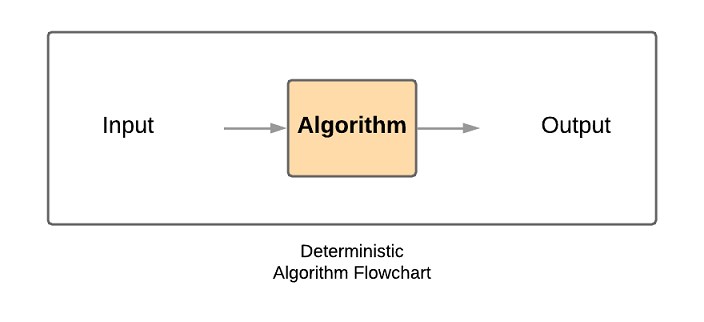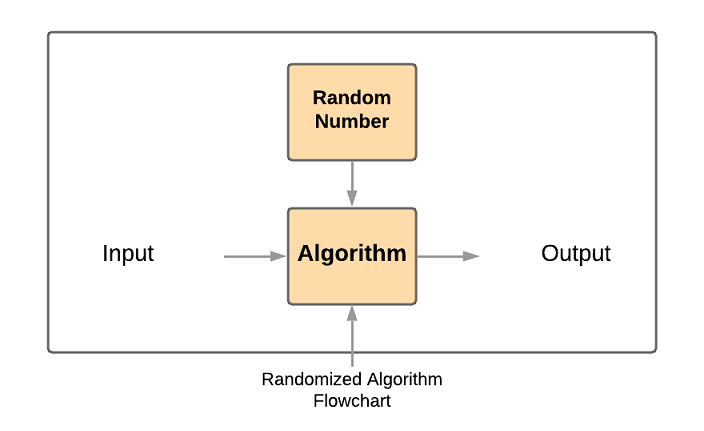×

Search anything:

# Understand Randomized Algorithms once and for all

#### Algorithms Randomized AlgorithmGet this book -> Problems on Array: For Interviews and Competitive Programming

Randomized algorithms use a certain amount of randomness as part of their logic. And counter-intuitively, the randomness can actually help us solve certain problems better than we could have otherwise! In this post, we discuss what randomized algorithms are, and have a look at the Solovay-Strassen Primality Tester to see what they are like.

# Introduction

Randomized algorithms use a source of randomness to make their decisions. They are used to reduce usage of resources such as time, space or memory by a standard algorithm.

A standard, deterministic algorithm will always produce the same output given a particular input. It will always pass through the same steps to reach that output, i.e. its behavior does not change on different runs. A real life example of this would be a known chemical reaction. Two parts hydrogen and one part oxygen will always make two molecules of water.

The goal of a deterministic algorithm is to always solve a problem correctly and quickly (in polynomial time). This is what a flow chart of its process looks like:Compare that to what one for a randomized algorithm looks like:Here, in addition to input, the algorithm uses random numbers to make random choices during execution. For the same input there can be different output, i.e. its behavior changes with different runs. These algorithms depend upon Random Number Generators.

Note that this is different from the probabilistic analysis of algorithms, wherein random input is used to show that the algorithm works for most inputs.

All of this may be too abstract. Let us look at an example of a randomized algorithm at work. Suppose one wants to find out the opinion of a large number of people on a particular topic. What would be the best way to do this?

Going to each and every person in such a survey is time consuming and would take ages to complete. Instead, taking a few people randomly and interviewing them would achieve the same. This is known as random sampling, and is an unbiased representation of a group. It is a fair way to select a sample from a larger population, since every member of the population has an equal chance of getting selected.

## When is randomness useful?

Another question that arises is- When are randomized algorithms used?

They are used whenever we are presented with time or memory constraints, and when an average case solution is acceptable. So you will see randomized algorithms in fields such as:

• Number theoretic algorithms – Primality Testing
• Data Structures – Searching, sorting, computational geometry
• Algebraic Identities – Verification of polynomial and matrix identities
• Mathematical Programming – Faster linear programming algorithms
• Graph algorithms – Finding minimum spanning trees, shortest paths, minimum cuts
• Counting and enumeration – Counting combinational structures
• Parallel and distributed computing – Deadlock avoidance, distributed consensus
• De-randomization - Randomness can be viewed as a resource, like space and time. De-randomization is then the process of removing randomness (or using as little of it as possible).

A very early introduction to the importance of algorithms is something you may have come across learning sorting algorithms, specifically - in Quicksort. The worst case for quick sort is to come across a list that is already mostly sorted (the time complexity shoots up to O(n2). Randomized Quicksort avoids this by shuffling the positions of the elements in the list, and on average Quicksort is able to sort in O(nlogn) time.

Since randomized algorithms have the potential of giving wrong outputs, amplification algorithms are used to boost the probability of correctness, at the expense of runtime. This can be done as long as the probability of an algorithm’s success is independent from run to run. Amplification works by running an algorithm many times (with different random subsamples of the input) and taking the majority answer. We shall use this property later when we make a primality tester.

# Random Number Generation

The various applications of randomness have led to the development of several different methods for generating random data, of which some have existed since ancient times, among whose ranks are well-known "classic" examples, including the rolling of dice,coin flipping, the shuffling of playing cards, as well as countless other techniques.

### Physical Methods

This involves actually relying on measurings of entropy from atomic and subatomic phenomena, whose unpredictability can be traced using laws of quantum mechanics. Sources of entropy include radioactive decay,thermal noise, avalanche noise in Zener diodes and radio noise.

Physical phenomena and tools used to measure them generally feature asymmetries and systematic biases that make their outcomes not uniformly random.

### Computational Methods

How are random numbers generated by machines? Machines are inherently deterministic in nature, so can they produce truly random numbers?

They cannot. Most computer generated random numbers use pseudorandom number generators(PRNGs) which are algorithms that can automatically create long runs of numbers with good random properties but eventually the sequence repeats (or the memory usage grows without bound). The series of values generated by such algorithms is generally determined by a fixed number called a "seed".

These numbers generated are not as random as those generated from electromagnetic or atmospheric noise used as a source of entropy.

# Las Vegas and Monte Carlo Algorithms

A Las Vegas algorithm is a randomized algorithm that always produces a correct result, or simply doesn’t find one, but it cannot guarantee a time constraint.The time complexity varies on the input. It does, however, guarantee an upper bound in the worst-case scenario.

In contrast to Las Vegas algorithms, a Monte Carlo algorithm is a probabilistic algorithm which, depending on the input, has a slight probability of producing an incorrect result or failing to produce a result altogether.This probability of incorrectness is known and is typically small.

Again, one must note how this is different from a deterministic algorithm, which will always give the correct output.

#### Trivia

The name of the algorithm comes from the grand casino in Monte Carlo, a world-wide icon of gambling.The term "Monte Carlo" was first introduced in 1947 by Nicholas Metropolis. Las Vegas algorithms were named in 1979 as a parallel to the Monte Carlo algorithm, as both are major gambling centres. In 1982, a third kind of randomized algorithm was discovered and was aptly called Atlantic City algorithm.

# The Solovay-Strassen Primality Test

A primality tester is an algorithm that checks whether a given integer is prime or not. This is a fairly common problem, especially in cyber security and cryptography, and many other applications that rely on the special properties of prime numbers.

This test was developed by Robert M. Solovay and Volker Strassen, and is a probabilistic test to determine if a number is composite or probably prime.

Before we dive in to the algorithm, a bit of mathematics and modular arithmetic terms need to be discussed:

## Key Concepts

### Legendre symbol (a/p):

It is a function defined on a pair of integers a and p such that-

(a/p) = 0; if a≡0(mod p)
(a/p) = 1; if k exists such that k^2 = a mod p exists
(a/p) = -1; otherwise

It follows Euler's criterion (see references), a fact we shall make use of later.

### Jacobi symbol (a/n):

It is a generalization of Legendre’s symbol. For any integeraand any positive odd integern, the Jacobi symbol(a/n)is defined as the product of the Legendre symbols corresponding to the prime factors of n:
(a/n) = (a/p1)k1.(a/p2)k2.(a/p3)k3...........(a/pn)kn

Where n = p1k1.p2k2......pnkn

Certain properties of the Jacobi symbol will become useful to us when it comes to finding out if a number is prime or not. For odd primes, (a/n) = (a/p).

The Jacobi symbol for a number can be computed in O((logn)²) time. For more properties, see references below.

# Algorithm for Solovay-Strassen Primality Test

The test works like this-

1. We first select a number n to test for its primality and a random number a which lies in the range of (2, n-1) and then compute the Jacobi symbol (a/n).
2. If n is a prime number then the Jacobi will be equal to the Legendre and it will satisfy Euler’s criterion.
3. If it does not satisfy the given condition then n is composite and the program will stop.
4. Like other probabilistic primality tests its accuracy is also directly proportional to the number of iterations. To get more accurate results, we shall run the test multiple times.

This random number a we took is called an Euler witness for n - it is a witness for the compositeness of n. Using fast algorithms for modular exponentiation, the running time of this algorithm is O (k·log3n), where k is the number of different values of a we test.

Here is the pseudo-code implementation of the Solovay-Strassen Primality Test:

## Psuedocode Implementation

``````# check if integer p is prime or not, run for k iterations
boolean SolovayStrassen(double p, int k):
if(p<2):
return false
if(p!=2 and p%2 == 0):
return false

for(int i = 0; i<k; i++):
double a = rand() % (p-1) + 1
# Jacobi() calculates the Jacobi symbol (a/n)
# modExp() performs modular exponentiation
double jacobian = (p + Jacobi(a, p)) % p
double mod = modExp(a, (p-1)/2, p)

if(!jacobian || mod != jacobian)
return false
return true
``````

# Wrapping up

Randomized algorithms use a certain amount of randomness as part of their logic. They have the ability to make random choices, allowing certain problems to be solved faster that otherwise require more time if solved deterministically.

Do we use properties like randomness in the field of Machine Learning?

Absolutely.
A lot of ML applications harness the power of randomness.
For example:

• An algorithm may be initialized to a random state, such as the initial weights in an artificial neural network.
• Internal decisions like voting/polling during training in a deterministic method may rely on randomness to resolve ties.
• the splitting of data into random train and test sets
• the random shuffling of a training dataset in stochastic gradient descent

Thus, generating random numbers and harnessing randomness is a required skill. Also, you may have noticed how your ML models return different results every time you run them - this is because of the degree of randomness involved. This random behavior within a range is called "stochastic" behaviour, and machine learning models are stochastic in practice.

We know that Monte Carlo algorithms are always fast and probably correct and Las Vegas algorithms are sometimes fast but always correct. Atlantic City algorithms lie in the middle of these two: it is almost always fast, and almost always correct. Designing these algorithms is an extremely complex process, thus very few of them are in existence.